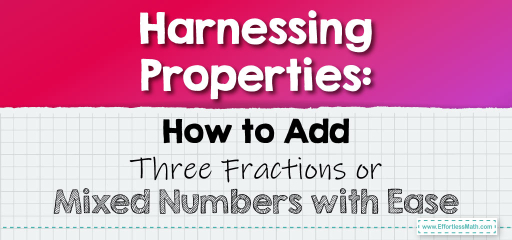# Harnessing Properties: How to Add Three Fractions or Mixed Numbers with Ease

Adding fractions or mixed numbers can sometimes be a challenge, especially when dealing with three or more.However, by utilizing the properties of addition, this process can be simplified and made more intuitive. In this guide, we’ll explore how to add three fractions or mixed numbers by leveraging these properties.

## Step-by-step Guide to Add Three Fractions or Mixed Numbers with Ease:

– Commutative Property: The order in which numbers are added doesn’t affect the sum. For example, $$a + b = b + a$$.

– Associative Property: The way numbers are grouped in addition doesn’t affect the sum. For example, $$a + (b + c) = (a + b) + c$$.

2. Converting Mixed Numbers to Improper Fractions:

If you’re dealing with mixed numbers, convert them to improper fractions. This makes the addition process more straightforward.

3. Finding the Least Common Denominator (LCD):

Determine the smallest number into which all the denominators can divide. This will ensure that the fractions are of comparable sizes.

4. Adjusting Each Fraction to the LCD:

Modify each fraction so that they all have the LCD as their denominator.

With the same denominator in place, simply add up all the numerators to get the final answer.

6. Converting Back to Mixed Numbers (if necessary):

If the result is an improper fraction, and you need a mixed number, convert it back.

### Example 1:

Add $$\frac{1}{4}$$, $$\frac{2}{8}$$, and $$1 \frac{1}{2}$$.

Solution:

First, convert the mixed number: $$1 \frac{1}{2}$$ becomes $$\frac{3}{2}$$.

The LCD for 4, 8, and 2 is 8. Adjusting the fractions:

– $$\frac{1}{4}$$ becomes $$\frac{2}{8}$$.

– $$\frac{2}{8}$$ remains the same.

– $$\frac{3}{2}$$ becomes $$\frac{12}{8}$$.

Adding them up, the result is $$\frac{16}{8}$$, which is equal to 2.

The Absolute Best Book for 5th Grade Students

### Example 2:

Add $$\frac{1}{3}$$, $$\frac{2}{6}$$, and $$2 \frac{1}{2}$$.

Solution:

First, convert the mixed number: $$2 \frac{1}{2}$$ becomes $$\frac{5}{2}$$.

The LCD for 3, 6, and 2 is 6. Adjusting the fractions:

– $$\frac{1}{3}$$ becomes $$\frac{2}{6}$$.

– $$\frac{2}{6}$$ remains the same.

– $$\frac{5}{2}$$ becomes $$\frac{15}{6}$$.

Adding them up, the result is $$\frac{19}{6}$$, which can be expressed as $$3 \frac{1}{6}$$.

### Practice Questions:

1. Add $$\frac{1}{5}$$, $$\frac{2}{10}$$, and $$1 \frac{3}{4}$$.

2. Add $$\frac{3}{7}$$, $$\frac{2}{14}$$, and $$2 \frac{1}{2}$$.

3. Add $$\frac{4}{9}$$, $$\frac{2}{3}$$, and $$3 \frac{1}{3}$$.

A Perfect Book for Grade 5 Math Word Problems!

1. $$2 \frac{9}{20}$$

2. $$4 \frac{1}{14}$$

3. $$6 \frac{5}{9}$$

The Best Math Books for Elementary Students

### What people say about "Harnessing Properties: How to Add Three Fractions or Mixed Numbers with Ease - Effortless Math: We Help Students Learn to LOVE Mathematics"?

No one replied yet.

X
51% OFF

Limited time only!

Save Over 51%

SAVE $15 It was$29.99 now it is \$14.99